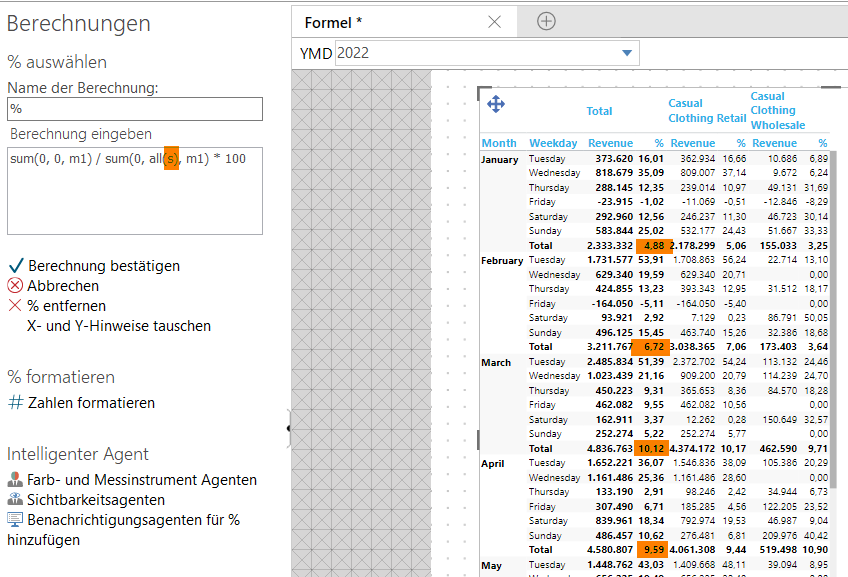# Calculation formula: Change Total of siblings

Hi all,

I added a calculation where I use the all(s) to have a %-value per month. That works fine!

But is there a way that my Total also makes a sum per month and shows 100 instead of 4,88?Thank you very much

• Hi Marlene

Yes there is - if you use the (c) modifier - which stands for "children", you can test if you are operating on a subtotal/total level, or you are working on a "leaf" - most granular level.

The standard syntax to determine which level you are working on looks something like this:

if allcount(d1,all(c),m1)>0 then something else something

The allcount will return a number greater than zero for all subtotals/totals and just zero when it comes to ordinary rows in the table.

So I guess your syntax could be something like this:

if allcount(d1, all(c), m1) > 0 then 1 else sum(d1, 0, m1) / sum(d1, all(s), m1)

br/Niels

• Thanks for the prompt help, the formula works!